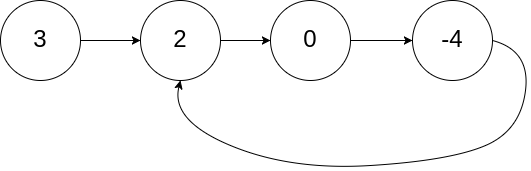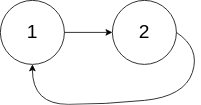## 刷题内容

Given a linked list, determine if it has a cycle in it.

To represent a cycle in the given linked list, we use an integer pos which represents the position (0-indexed) in the linked list where tail connects to. If pos is -1, then there is no cycle in the linked list.


Example 1:

Input: head = [3,2,0,-4], pos = 1
Output: true
Explanation: There is a cycle in the linked list, where tail connects to the second node.Example 2:

Input: head = [1,2], pos = 0
Output: true
Explanation: There is a cycle in the linked list, where tail connects to the first node.Example 3:

Input: head = , pos = -1
Output: false
Explanation: There is no cycle in the linked list.Can you solve it using O(1) (i.e. constant) memory?

## 解题方案

#### 执行结果

/**
* function ListNode(val) {
*     this.val = val;
*     this.next = null;
* }
*/

/**
* @return {boolean}
*/
return false
}